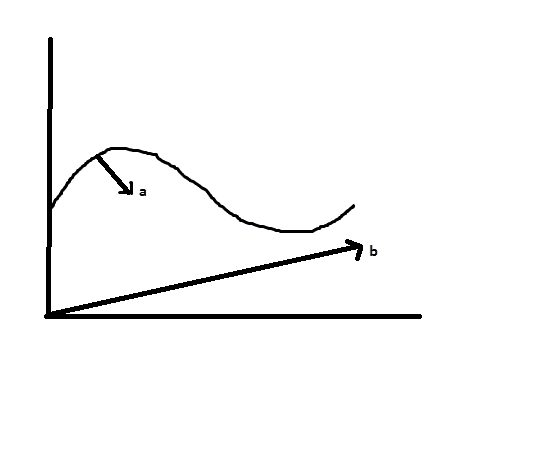# Projectiles and Vectors Graphs

• physicsnobrain
Certainly, but then we aren't talking about the case of projectile motion, then it's just simple kinematics :-)

#### physicsnobrain

I have a theory question I was wondering about. Today we learned that on a graph of projectile motion, velocity always acts tangent to the graph of the projectile. Why is is this?

Also, does acceleration always act down on a projectile? How do acceleration and displacement vectors act on a projectile?

Thanks

Well, the velocity always have the direction of motion. http://en.wikipedia.org/wiki/File:Ferde_hajitas2.svg
This illustration from wikipedia actually pictures it very well. The x and y components of the velocity change as the ball moves along the line. The obvious relationship between them, determines the direction of the projectile. In the start, the y component is larger than the x, since it moves more upwards then sideways, but when reaches the middle, the x component get larger, and the y component drops. This relationship ensures that it is always tangential to the curve.

Yes, acceleration is always downwards the y-axis, if you neglect air resistance. The acceleration is due to gravity, and is always constant. You use this to determine the top point, your projectile can reach, before it starts falling. Since the acceleration is downwards, it makes the projectile lose more and more velocity as it rises.

I am not sure, what you mean with displacement vectors? A displacement vector points from your starting position to wherever the projectile is, as far as I am concerned.

So in this sort of graph then b would represent displacement because it is measuring distance from 0 and a would be acceleration because it is downward right?physicsnobrain said:
So in this sort of graph then b would represent displacement because it is measuring distance from 0 and a would be acceleration because it is downward right?this is a graph of the movement of a particle btw.

There is no acceleration in the x-direction. The acceleration is only in the y-direction, it has to point in the same direction as the y-axis. This is important, since if it didn't, what I explained above with the relationship between the x and y component of the acceleration, would not hold. At least, when you are talking projectile motion.

For the particle you show, it depends if it can accelerate on its own. Also don't confuse "particle" with anything small, since these laws actually don't hold for very small particles such as molecules and atoms. Do not think about it, just keep it in mind, since a lot of physics teachers make mistakes in these parts.

hjelmgart said:
There is no acceleration in the x-direction. The acceleration is only in the y-direction, it has to point in the same direction as the y-axis. This is important, since if it didn't, what I explained above with the relationship between the x and y component of the acceleration, would not hold. At least, when you are talking projectile motion.

For the particle you show, it depends if it can accelerate on its own. Also don't confuse "particle" with anything small, since these laws actually don't hold for very small particles such as molecules and atoms. Do not think about it, just keep it in mind, since a lot of physics teachers make mistakes in these parts.

Well judging off that graph I believe it can accelerate on its own.

physicsnobrain said:
Well judging off that graph I believe it can accelerate on its own.

Certainly, but then we aren't talking about the case of projectile motion, then it's just simple kinematics :-) but what it means, is that the particle can accelerate on its own.